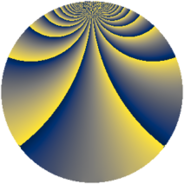# Properties

 Label 1694.2.rLevel $1694$ Weight $2$ Character orbit 1694.r Rep. character $\chi_{1694}(215,\cdot)$ Character field $\Q(\zeta_{30})$ Dimension $576$ Sturm bound $528$

# Related objects

## Defining parameters

 Level: $$N$$ $$=$$ $$1694 = 2 \cdot 7 \cdot 11^{2}$$ Weight: $$k$$ $$=$$ $$2$$ Character orbit: $$[\chi]$$ $$=$$ 1694.r (of order $$30$$ and degree $$8$$) Character conductor: $$\operatorname{cond}(\chi)$$ $$=$$ $$77$$ Character field: $$\Q(\zeta_{30})$$ Sturm bound: $$528$$

## Dimensions

The following table gives the dimensions of various subspaces of $$M_{2}(1694, [\chi])$$.

Total New Old
Modular forms 2304 576 1728
Cusp forms 1920 576 1344
Eisenstein series 384 0 384

## Trace form

 $$576q - 72q^{4} - 12q^{5} + 10q^{7} - 84q^{9} + O(q^{10})$$ $$576q - 72q^{4} - 12q^{5} + 10q^{7} - 84q^{9} + 2q^{14} + 12q^{15} + 72q^{16} + 30q^{17} + 16q^{23} - 60q^{25} + 24q^{26} - 10q^{28} - 20q^{29} + 18q^{31} - 30q^{35} - 128q^{36} - 12q^{37} + 12q^{38} - 20q^{39} + 30q^{40} - 6q^{42} - 108q^{45} + 36q^{47} + 82q^{49} + 60q^{51} - 12q^{53} - 4q^{56} + 80q^{57} + 32q^{58} + 60q^{59} - 4q^{60} - 30q^{61} - 50q^{63} + 144q^{64} + 48q^{67} - 30q^{68} + 18q^{70} + 8q^{71} - 20q^{72} - 90q^{73} - 20q^{74} - 156q^{75} - 160q^{78} - 30q^{79} - 18q^{80} + 40q^{81} - 84q^{82} - 40q^{84} - 140q^{85} - 2q^{86} - 12q^{89} + 46q^{91} - 28q^{92} - 30q^{93} + 120q^{94} + 70q^{95} + O(q^{100})$$

## Decomposition of $$S_{2}^{\mathrm{new}}(1694, [\chi])$$ into newform subspaces

The newforms in this space have not yet been added to the LMFDB.

## Decomposition of $$S_{2}^{\mathrm{old}}(1694, [\chi])$$ into lower level spaces

$$S_{2}^{\mathrm{old}}(1694, [\chi]) \cong$$ $$S_{2}^{\mathrm{new}}(77, [\chi])$$$$^{\oplus 4}$$$$\oplus$$$$S_{2}^{\mathrm{new}}(154, [\chi])$$$$^{\oplus 2}$$$$\oplus$$$$S_{2}^{\mathrm{new}}(847, [\chi])$$$$^{\oplus 2}$$